Python机器学习笔记：随机森林算法

完整代码及其数据，请移步小编的GitHub

传送门：请点击我

如果点击有误：https://github.com/LeBron-Jian/MachineLearningNote

随机森林算法的理论知识

随机森林是一种有监督学习算法，是以决策树为基学习器的集成学习算法。随机森林非常简单，易于实现，计算开销也很小，但是它在分类和回归上表现出非常惊人的性能，因此，随机森林被誉为“代表集成学习技术水平的方法”。

一，随机森林的随机性体现在哪几个方面？

1，数据集的随机选取

从原始的数据集中采取有放回的抽样（bagging），构造子数据集，子数据集的数据量是和原始数据集相同的。不同子数据集的元素可以重复，同一个子数据集中的元素也可以重复。

2，待选特征的随机选取

与数据集的随机选取类似，随机森林中的子树的每一个分裂过程并未用到所有的待选特征，而是从所有的待选特征中随机选取一定的特征，之后再在随机选取的特征中选取最优的特征

二，为什么使用随机森林？

1，随机森林既可以用于分类问题，也可以用于回归问题

2，过拟合是个关键的问题，可能会让模型的结果变得糟糕，但是对于随机森林来说，如果随机森林的树足够多，那么分类器就不会过拟合模型

3，随机森林分类器可以处理缺失值

4，随机森林分类器可以用分类值建模

三，随机森林的构建过程

1，从原始训练集中使用Bootstraping方法随机有放回采样取出m个样本，共进行n_tree次采样。生成n_tree个训练集

2，对n_tree个训练集，我们分别训练n_tree个决策树模型

3，对于单个决策树模型，假设训练样本特征的个数为n，那么每次分裂时根据信息增益/信息增益比/基尼指数  选择最好的特征进行分裂

4，每棵树都已知这样分裂下去，知道该节点的所有训练样例都属于同一类。在决策树的分裂过程中不需要剪枝

5，将生成的多颗决策树组成随机森林。对于分类问题，按照多棵树分类器投票决定最终分类结果；对于回归问题，由多颗树预测值的均值决定最终预测结果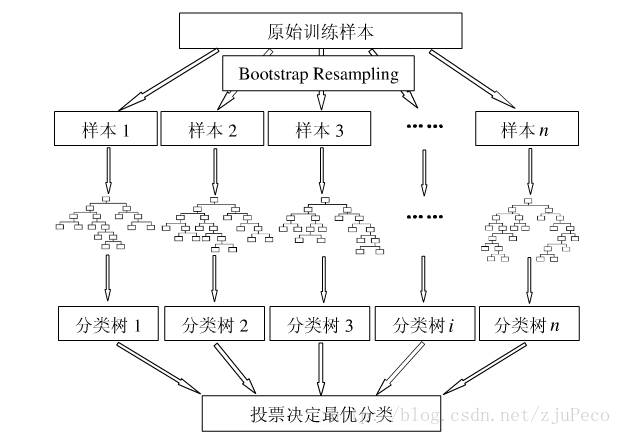四，随机森林优缺点总结

优点

• 由于采用了集成算法，本身精度比大多数单个算法要好，所以准确性高
• 在测试集上表现良好，由于两个随机性的引入，使得随机森林不容易陷入过拟合（样本随机，特征随机）
• 在工业上，由于两个随机性的引入，使得随机森林具有一定的抗噪声能力，对比其他算法具有一定优势
• 由于树的组合，使得随机森林可以处理非线性数据，本身属于非线性分类（拟合）模型
• 它能够处理很高维度（feature很多）的数据，并且不用做特征选择，对数据集的适应能力强：既能处理离散型数据，也能处理连续型数据，数据集无需规范化
• 训练速度快，可以运用在大规模数据集上
• 可以处理缺省值（单独作为一类），不用额外处理
• 由于有袋外数据（OOB），可以在模型生成过程中取得真实误差的无偏估计，且不损失训练数据量
• 在训练过程中，能够检测到feature间的互相影响，且可以得出feature的重要性，具有一定参考意义
• 由于每棵树可以独立、同时生成，容易做成并行化方法
• 由于实现简单、精度高、抗过拟合能力强，当面对非线性数据时，适于作为基准模型

缺点

• 当随机森林中的决策树个数很多时，训练时需要的空间和时间会比较大
• 随机森林中还有许多不好解释的地方，有点算是黑盒模型
• 在某些噪音比较大的样本集上，RF的模型容易陷入过拟合

五，特征重要性评估

现实情况下，一个数据集中往往有成百上千个特征，如何在其中选择比结果影响最大的那几个特征，以此来缩减建立模型时特征数是我们比较关心的问题。这样的方法其实很多，比如主成分分析，lasso等等。不过这里我们学习的是用随机森林来进行特征筛选。

用随机森林进行特征重要性评估的思想就是看每个特征在随机森林中的每棵树上做了多大的贡献，然后取个平均值，最后比一比特征之间的贡献大小。

贡献大小通常使用基尼指数（Gini index）或者袋外数据（OOB）错误率作为评估指标来衡量。这里我们再学习一下基尼指数来评价的方法。

我们将变量重要性评分（variable importance measures）用VIM来表示，将Gini指数用GI来表示，假设m个特征X1，X2，X3，......Xc，现在要计算出每个特征Xj的Gini指数评分VIMj（Gini）,亦即第j个特征在RF所有决策树中节点分裂不纯度的平均改变量。

Gini指数的计算公式为：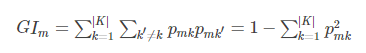其中，K表示有K个类别。Pmk表示节点m中类列k所占的比例。

直观的说，就是随便从节点m中随机抽取两个样本，其类别标记不一致的概率。

特征Xj在节点m的重要性，即节点m分支前后的Gini指数变化量为：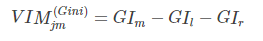其中，GIl和GIr分别表示分枝后两个新节点的Gini指数。

如果，特征Xj在决策树i中出现的节点在集合M中，那么Xj在第i颗树的重要性为：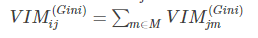假设RF中共有n颗树，那么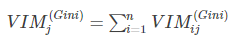最后，把所有求得的重要性评分做一个归一化处理即可。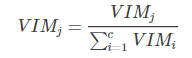5.1 特征选择

5.1.1 特征选择的步骤

在特征重要性的基础上，特征选择的步骤如下：

• 计算每个特征的重要性，并按降序排序
• 确定要剔除的比例，依据特征重要性剔除相应比例的特征，得到一个新的特征集
• 用新的特征集重复上述过程，直到剩下m个特征（m为提前设定的值）
• 根据上述代码中得到的各个特征集合特征集对应的袋外误差率，选择袋外误差率最低的特征集

5.1.2 特征重要性的估计方法

特征重要性的估计通常有两种方法：一是使用uniform或者gaussian抽取随机值替换原特征；一是通过permutation的方式将原来的所有N个样本的第i个特征值重新打乱分布，第二种方法更加科学，保证了特征替代值与原特征的分布是近似的。这种方法叫做permutation test ，即在计算第i个特征的重要性的时候，将N 个特征的第i个特征重新洗牌，然后比较D和表现的差异性，如果差异很大，则表明第i个特征是重要的。

5.2  示例——利用随机森林进行特征选择，然后使用SVR进行训练

1，利用随机森林进行特征选择

代码：

import numpy as np
import pandas as pd
from sklearn.ensemble import RandomForestClassifier

# url = 'http://archive.ics.uci.edu/ml/machine-learning-databases/wine/wine.data'
# url1 = pd.DataFrame(url1)
url1.columns = ['Class label', 'Alcohol', 'Malic acid', 'Ash',
'Alcalinity of ash', 'Magnesium', 'Total phenols',
'Flavanoids', 'Nonflavanoid phenols', 'Proanthocyanins',
'Color intensity', 'Hue', 'OD280/OD315 of diluted wines', 'Proline']

# 重要性： [0.10658906 0.02539968 0.01391619 0.03203319 0.02207807 0.0607176
#  0.15094795 0.01464516 0.02235112 0.18248262 0.07824279 0.1319868
#  0.15860977]
# print(url1)

# 查看几个标签
# Class_label = np.unique(url1['Class label'])
# print(Class_label)
# 查看数据信息
# info_url = url1.info()
# print(info_url)

# 除去标签之外，共有13个特征，数据集的大小为178，
# 下面将数据集分为训练集和测试集
from sklearn.model_selection import train_test_split

print(type(url1))
# url1 = url1.values
# x = url1[:,0]
# y = url1[:,1:]
x, y = url1.iloc[:, 1:].values, url1.iloc[:, 0].values
x_train, x_test, y_train, y_test = train_test_split(x, y, test_size=0.3, random_state=0)
feat_labels = url1.columns[1:]
# n_estimators：森林中树的数量
# n_jobs  整数 可选（默认=1） 适合和预测并行运行的作业数，如果为-1，则将作业数设置为核心数
forest = RandomForestClassifier(n_estimators=10000, random_state=0, n_jobs=-1)
forest.fit(x_train, y_train)

# 下面对训练好的随机森林，完成重要性评估
# feature_importances_  可以调取关于特征重要程度
importances = forest.feature_importances_
print("重要性：", importances)
x_columns = url1.columns[1:]
indices = np.argsort(importances)[::-1]
x_columns_indices = []
for f in range(x_train.shape):
# 对于最后需要逆序排序，我认为是做了类似决策树回溯的取值，从叶子收敛
# 到根，根部重要程度高于叶子。
print("%2d) %-*s %f" % (f + 1, 30, feat_labels[indices[f]], importances[indices[f]]))
x_columns_indices.append(feat_labels[indices[f]])

print(x_columns_indices)
print(x_columns.shape)
print(x_columns)
print(np.arange(x_columns.shape))

# 筛选变量（选择重要性比较高的变量）
threshold = 0.15
x_selected = x_train[:, importances > threshold]

# 可视化
import matplotlib.pyplot as plt

plt.figure(figsize=(10, 6))
plt.title("红酒的数据集中各个特征的重要程度", fontsize=18)
plt.ylabel("import level", fontsize=15, rotation=90)
plt.rcParams['font.sans-serif'] = ["SimHei"]
plt.rcParams['axes.unicode_minus'] = False
for i in range(x_columns.shape):
plt.bar(i, importances[indices[i]], color='orange', align='center')
plt.xticks(np.arange(x_columns.shape), x_columns_indices, rotation=90, fontsize=15)
plt.show()

结果：

RangeIndex: 178 entries, 0 to 177
Data columns (total 14 columns):
Class label                     178 non-null int64
Alcohol                         178 non-null float64
Malic acid                      178 non-null float64
Ash                             178 non-null float64
Alcalinity of ash               178 non-null float64
Magnesium                       178 non-null int64
Total phenols                   178 non-null float64
Flavanoids                      178 non-null float64
Nonflavanoid phenols            178 non-null float64
Proanthocyanins                 178 non-null float64
Color intensity                 178 non-null float64
Hue                             178 non-null float64
OD280/OD315 of diluted wines    178 non-null float64
Proline                         178 non-null int64
dtypes: float64(11), int64(3)
memory usage: 19.5 KB

0.15094795 0.01464516 0.02235112 0.18248262 0.07824279 0.1319868
0.15860977]

1) Color intensity                0.182483
2) Proline                        0.158610
3) Flavanoids                     0.150948
4) OD280/OD315 of diluted wines   0.131987
5) Alcohol                        0.106589
6) Hue                            0.078243
7) Total phenols                  0.060718
8) Alcalinity of ash              0.032033
9) Malic acid                     0.025400
10) Proanthocyanins                0.022351
11) Magnesium                      0.022078
12) Nonflavanoid phenols           0.014645
13) Ash                            0.013916

图：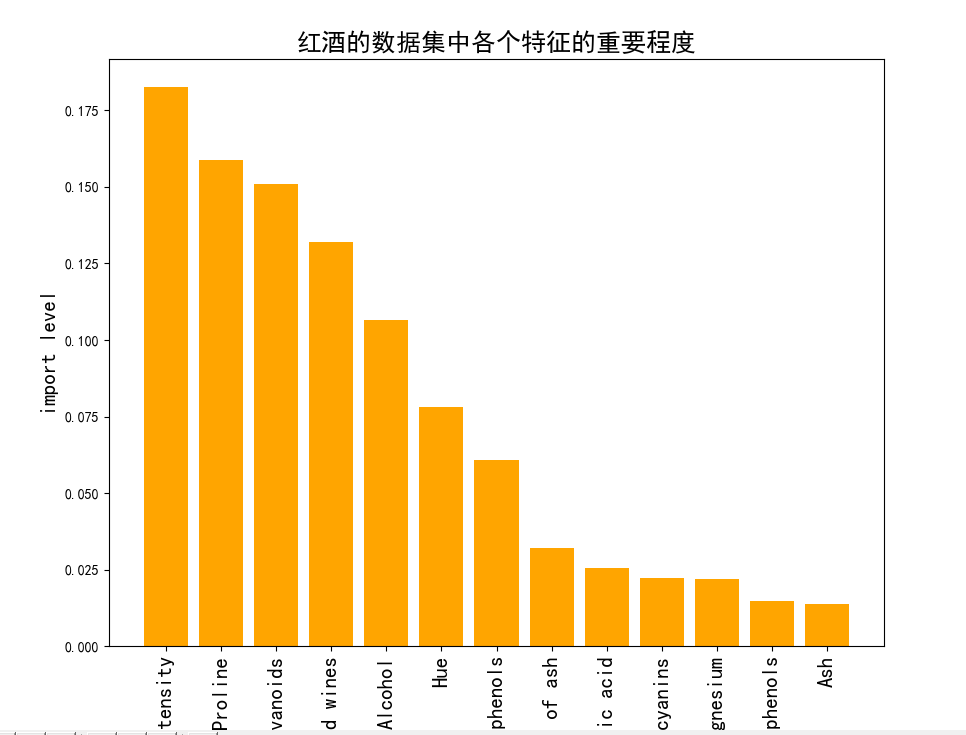2，利用SVR进行训练

代码：

from sklearn.svm import SVR  # SVM中的回归算法
import pandas as pd
from sklearn.model_selection import train_test_split
import matplotlib.pyplot as plt
import numpy as np
# 数据预处理，使得数据更加有效的被模型或者评估器识别
from sklearn import preprocessing
from sklearn.externals import joblib

# 获取数据
X = origin_data.iloc[:,1:].values
Y = origin_data.iloc[:,0].values
print(type(Y))
# print(type(Y.values))
# 总特征  按照特征的重要性排序的所有特征
all_feature = [ 9, 12,  6, 11,  0, 10,  5,  3,  1,  8,  4,  7,  2]
# 这里我们选取前三个特征
topN_feature = all_feature[:3]
print(topN_feature)

# 获取重要特征的数据
data_X = X[:,topN_feature]

# 将每个特征值归一化到一个固定范围
# 原始数据标准化，为了加速收敛
# 最小最大规范化对原始数据进行线性变换，变换到[0,1]区间
data_X = preprocessing.MinMaxScaler().fit_transform(data_X)

# 利用train_test_split 进行训练集和测试集进行分开
X_train,X_test,y_train,y_test  = train_test_split(data_X,Y,test_size=0.3)

# 通过多种模型预测
model_svr1 = SVR(kernel='rbf',C=50,max_iter=10000)

# 训练
# model_svr1.fit(data_X,Y)
model_svr1.fit(X_train,y_train)

# 得分
score = model_svr1.score(X_test,y_test)
print(score)

结果：

0.8211850237886935

scikit-learn随机森林类库概述

sklearn.ensemble模块包含了两种基于随机决策树的平均算法：RandomForest算法和Extra-Trees算法。这两种算法都采用了很流行的树设计思想：perturb-and-combine思想。这种方法会在分类器的构建时，通过引入随机化，创建一组各不一样（diverse）的分类器。这种ensemble方法的预测会给出各个分类器预测的平均。

在sklearn.ensemble库中，我们可以找到Random Forest分类和回归的实现：RandomForestClassifier和RandomForestRegression 有了这些模型后，我们的做法是立马上手操作，因为学习中提供的示例都很简单，但是实际中遇到很多问题，下面概述一下：

• 命名模型调教的很好了，可是效果离我们的想象总有些偏差？——模型训练的第一步就是要定要目标，往错误的方向走太多也是后退。
• 凭直觉调了某个参数，可是居然没有任何作用，有时甚至起到反作用？——定好目标后，接下来就是要确定哪些参数是影响目标的，其对目标是正影响还是负影响，影响的大小。
• 感觉训练结束遥遥无期，sklearn只是一个在小数据上的玩具？——虽然sklearn并不是基于分布式计算环境而设计的，但是我们还是可以通过某些策略提高训练的效率
• 模型开始训练了，但是训练到哪一步了呢？——饱暖思淫欲啊，目标，性能和效率都得了满足后，我们有时还需要有别的追求，例如训练过程的输出，袋外得分计算等等。

在scikit-learn中，RF的分类类是RandomForestClassifier，回归类是RandomForestRegressor。当然RF的变种Extra Trees也有，分类类ExtraTreesClassifier，回归类ExtraTreesRegressor。由于RF和Extra Trees的区别较小，调参方法基本相同，本文只关注于RF的调参。

RandomForests

在随机森林（RF）中，该ensemble方法中的每棵树都基于一个通过可放回抽样（boostrap）得到的训练集构建。另外，在构建树的过程中，当split一个节点时，split的选择不再是对所有features的最佳选择。相反的，在features的子集中随机进行split反倒是最好的split方式。这种随机的后果是，整个forest的bias，从而得到一个更好的模型。

sklearn的随机森林（RF）实现通过对各分类结果预测求平均得到，而非让每个分类器进行投票（vote）。

Ext-Trees

在Ext-Trees中(详见ExtraTreesClassifier和 ExtraTreesRegressor)，该方法中，随机性在划分时会更进一步进行计算。在随机森林中，会使用侯选feature的一个随机子集，而非查找最好的阈值，对于每个候选feature来说，阈值是抽取的，选择这种随机生成阈值的方式作为划分原则。通常情况下，在减小模型的variance的同时，适当增加bias是允许的。

首先看一个类的参数：

class sklearn.ensemble.RandomForestClassifier(n_estimators=10, criterion='gini',
max_depth=None, min_samples_split=2, min_samples_leaf=1,
min_weight_fraction_leaf=0.0, max_features=’auto’, max_leaf_nodes=None,
min_impurity_decrease=0.0, min_impurity_split=None, bootstrap=True,
oob_score=False, n_jobs=1, random_state=None, verbose=0, warm_start=False, class_weight=None)

一，RF Bagging框架参数

和GBDT对比，GBDT的框架参数比较多，重要的有最大迭代器个数，步长和子采样比例，调参起来比较费力。但是RF则比较简单，这是因为Bagging框架里的各个弱学习器之间是没有依赖关系的，这减小调参的难度，换句话说，达到同样的调参效果，RF调参数时间要比GBDT少一些，

下面我来看看RF重要的Bagging框架的参数，由于RandomForestClassifier和RandomForestRegressor参数绝大部分相同，这里会将它们一起讲，不同点会指出。

1) n_estimators: 也就是弱学习器的最大迭代次数，或者说最大的弱学习器的个数。一般来说n_estimators太小，容易欠拟合，n_estimators太大，又容易过拟合，一般选择一个适中的数值。RandomForestClassifier和RandomForestRegressor默认是10。在实际调参的过程中，我们常常将n_estimators和下面介绍的参数learning_rate一起考虑。

2) oob_score :即是否采用袋外样本来评估模型的好坏。默认识False。有放回采样中大约36.8%的没有被采样到的数据，我们常常称之为袋外数据（Out Of Bag  简称OOB），这些数据没有参与训练集模型的拟合，因此可以用来检测模型的泛化能力。个人推荐设置为True，因为袋外分数反应了一个模型拟合后的泛化能力。

3) criterion: 即CART树做划分时对特征的评价标准。分类模型和回归模型的损失函数是不一样的。分类RF对应的CART分类树默认是基尼系数gini,另一个可选择的标准是信息增益。回归RF对应的CART回归树默认是均方差mse，另一个可以选择的标准是绝对值差mae。一般来说选择默认的标准就已经很好的。

4）bootstrap：默认是True，是否有放回的采样。

5）verbose：日志亢长度，int表示亢长度，o表示输出训练过程，1表示偶尔输出 ，>1表示对每个子模型都输出

从上面可以看出， RF重要的框架参数比较少，主要需要关注的是 n_estimators，即RF最大的决策树个数。当使用这些方法的时候，最主要的参数是调整n_estimators和max_features。n_estimators指的是森林中树的个数，树数目越大越好，但是会增加计算开销，另外，注意如果超过限定数量后，计算将会停止。

二，RF决策树参数

下面我们再来看RF的决策树参数，它要调参的参数基本和GBDT相同，如下：

1) RF划分时考虑的最大特征数max_features: 可以使用很多种类型的值，默认是"None",意味着划分时考虑所有的特征数；如果是"log2"意味着划分时最多考虑个特征；如果是"sqrt"或者"auto"意味着划分时最多考虑个特征。如果是整数，代表考虑的特征绝对数。如果是浮点数，代表考虑特征百分比，即考虑（百分比xN）取整后的特征数。其中N为样本总特征数。一般来说，如果样本特征数不多，比如小于50，我们用默认的"None"就可以了，如果特征数非常多，我们可以灵活使用刚才描述的其他取值来控制划分时考虑的最大特征数，以控制决策树的生成时间。max_features指的是，当划分一个节点的时候，features的随机子集的size，该值越小，variance会变小，但是bais会变大。（int 表示个数，float表示占所有特征的百分比，auto表示所有特征数的开方，sqrt表示所有特征数的开放，log2表示所有特征数的log2值，None表示等于所有特征数）

2) 决策树最大深度max_depth: 默认可以不输入，如果不输入的话，决策树在建立子树的时候不会限制子树的深度。一般来说，数据少或者特征少的时候可以不管这个值。如果模型样本量多，特征也多的情况下，推荐限制这个最大深度，具体的取值取决于数据的分布。常用的可以取值10-100之间。（int表示深度，None表示树会生长到所有叶子都分到一个类，或者某节点所代表的样本已小于min_samples_split）

3) 内部节点再划分所需最小样本数min_samples_split: 这个值限制了子树继续划分的条件，如果某节点的样本数少于min_samples_split，则不会继续再尝试选择最优特征来进行划分。 默认是2.如果样本量不大，不需要管这个值。如果样本量数量级非常大，则推荐增大这个值。（int表示样本数，2表示默认值）

4) 叶子节点最少样本数min_samples_leaf: 这个值限制了叶子节点最少的样本数，如果某叶子节点数目小于样本数，则会和兄弟节点一起被剪枝。 默认是1,可以输入最少的样本数的整数，或者最少样本数占样本总数的百分比。如果样本量不大，不需要管这个值。如果样本量数量级非常大，则推荐增大这个值。

5）叶子节点最小的样本权重和min_weight_fraction_leaf：这个值限制了叶子节点所有样本权重和的最小值，如果小于这个值，则会和兄弟节点一起被剪枝。 默认是0，就是不考虑权重问题。一般来说，如果我们有较多样本有缺失值，或者分类树样本的分布类别偏差很大，就会引入样本权重，这时我们就要注意这个值了。

6) 最大叶子节点数max_leaf_nodes: 通过限制最大叶子节点数，可以防止过拟合，默认是"None”，即不限制最大的叶子节点数。如果加了限制，算法会建立在最大叶子节点数内最优的决策树。如果特征不多，可以不考虑这个值，但是如果特征分成多的话，可以加以限制，具体的值可以通过交叉验证得到。

7) 节点划分最小不纯度min_impurity_split:  这个值限制了决策树的增长，如果某节点的不纯度(基于基尼系数，均方差)小于这个阈值，则该节点不再生成子节点。即为叶子节点 。一般不推荐改动默认值1e-7。

8）用于拟合和预测的并行运行的工作数量n_jobs：一般取整数，可选的（默认值为1），如果为-1，那么工作数量被设置为核的数量，机器上所有的核都会被使用（跟CPU核数一致）。如果n_jobs=k，则计算被划分为k个job，并运行在K核上。注意，由于进程间通信的开销，加速效果并不会是线性的（job数K不会提示K倍）通过构建大量的树，比起单颗树所需要的时间，性能也能得到很大的提升，

9）随机数生成器random_state：随机数生成器使用的种子，如果是RandomState实例，则random_stats就是随机数生成器；如果为None，则随机数生成器是np.random使用的RandomState实例。

上面决策树参数中最重要的包括最大特征数max_features， 最大深度max_depth， 内部节点再划分所需最小样本数min_samples_split和叶子节点最少样本数min_samples_leaf。

根据经验

对于回归问题：好的缺省值max_features = n_features;

对于分类问题：好的缺省值是max_features=sqrt(n_features)。n_features指的是数据中的feature总数。

当设置max_depth=None，以及min_samples_split=1时，通常会得到好的结果（完全展开的树）。但需要注意，这些值通常不是最优的，并且会浪费RAM内存。最好的参数应通过cross-validation给出。另外需要注意：

在随机森林中，缺省时会使用bootstrap进行样本抽样(bootstrap=True) ；
而extra-trees中，缺省策略为不使用bootstrap抽样 (bootstrap=False)；
当使用bootstrap样本时，泛化误差可能在估计时落在out-of-bag样本中。此时，可以通过设置oob_score=True来开启。

三，如何调参呢？

参数分类的目的在于缩小调参的范围，首先我们要明确训练的目标，把目标类的参数定下来。接下来，我们需要根据数据集的大小，考虑是否采用一些提高训练效率的策略，否则一次训练就三天三夜，时间太久了，所以我们需要调整哪些影响整体的模型性能的参数。

1，调参的目标：偏差和方差的协调

偏差和方差通过准确率来影响着模型的性能。调参的目标就是为了达到整体模型的偏差和方差的大和谐！进一步，这些参数又可以分为两类：过程影响类及子模型影响类。在子模型不变的前提下，某些参数可以通过改变训练的过程，从而影响着模型的性能，诸如：“子模型数”（n_estimators），“学习率”（learning_rate）等，另外，我们还可以通过改变子模型性能来影响整体模型的性能，诸如：“最大树深度”（max_depth），‘分裂条件’（criterion）等。正由于bagging的训练过程旨在降低方差，而Boosting的训练过程旨在降低偏差，过程影响类的参数能够引起整体模型性能的大幅度变化。一般来说，在此前提下，我们继续微调子模型影响类的参数，从而进一步提高模型的性能。

2，参数对整体模型性能的影响

假设模型是一个多元函数F，其输出值为模型的准确度。我们可以固定其他参数，从而对某个参数整体模型性能的影响进行分析：是正影响还是负影响，影响的单调性？

对Random Forest来说，增加“子模型树”（n_estimators）可以明显降低整体模型的方差，且不会对子模型的偏差和方差有任何影响。模型的准确度会随着“子模型数”的增加而提高，由于减少的是整体模型方差公式的第二项，故准确度的提高有一个上线。在不同的场景下，“分裂条件”（criterion）对模型的准确度的影响也不一样，该参数需要在实际运行时灵活调整。调整“最大叶子节点数”（max_leaf_models）以及“最大树深度”（max_depth）之一，可以粗粒度地调整树的结构：叶节点越多或者树越深，意味着子模型的偏差月底，方差越高；同时，调整”分裂所需要最小样本数”（min_samples_split），“叶节点最小样本数”（min_samples_leaf）及“叶节点最小权重总值”（min_weight_fraction_leaf），可以更细粒度地调整树的结构：分裂所需样本数越少或者叶节点所需样本越少，也意味着子模型越复杂。一般来说，我们总采用bootstrap对样本进行子采样来降低子模型之间的关联度，从而降低整体模型的方差。适当地减少“分裂时考虑的最大特征数”（max_features），给子模型注入了另外的随机性，同样也达到了降低子模型之间关联度的效果。但是一味地降低该参数也是不行的，因为分裂时可选特征变少，模型的偏差会越来越大。在下图中，我们可以看到这些参数对Random Forest整体模型性能的影响：3，一个朴实的方案：贪心的坐标下降法

到此为止，我们终于知道需要调整哪些参数，对于单个参数，我们也知道怎么调整才能提升性能。然后，表示模型的函数F并不是一元函数，这些参数需要共同调参才能得到全局最优解。也就是说，把这些参数丢给调参算法（诸如Grid Search）？对于小数据集，我们还能这么任性，但是参数组合爆炸，在大数据集上，实际上网格搜索也不一定能得到全局最优解。

坐标下降法是一类优化算法，其最大的优势在于不同计算待优化的目标函数的梯度。我们最容易想到一种特别朴实的类似于坐标下降法的方法，与坐标下降法不同的是，其不同循环使用各个参数进行调整，而是贪心地选取了对整体模型性能影响最大的参数。参数对整体模型性能的影响力是动态变化的，故每一轮坐标选取的过程中，这种方法在对每个坐标的下降方向进行一次直线搜索（line search）。首先，找到那些能够提升整体模型性能的参数，其次确保提升是单调或者近似单调。这意味着，我们筛选出来的参数是整体模型性能有正影响的，且这种影响不是偶然性的，要知道，训练过程的随机性也会导致整体模型性能的细微区别，而这种区别是不具有单调性的。最后，在这些筛选出来的参数中，选取影响最大的参数进行调整即可。

无法对整体模型性能进行量化，也就谈不上去比较参数影响整体模型性能的程度，是的，我们还没有一个准确的方法来量化整体模型性能，只能通过交叉验证来近似计算整体模型性能。然而交叉验证也存在随机性，假设我们以验证集上的平均准确度作为整体模型的准确度，我们还得关心在各个验证集上准确度的变异系数，如果变异系数过大，则平均值作为整体模型的准确率也是不合适的。在接下来的案例分析中，我们所谈及的整体模型性能均是指平均准确度。

4，调参步骤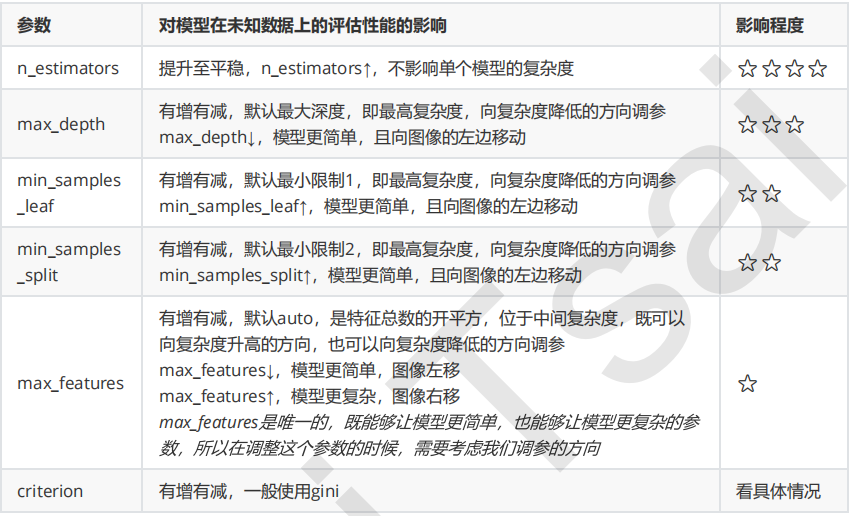• 1，首先先调既不会增加模型复杂度，又对模型影响最大的参数n_estimators（学习曲线）
• 2，找到最佳值后，调max_depth（单个网格搜索，也可以使用学习曲线）
• 　　一般根据数据的大小来进行一个探视，当数据集很小的时候，可以采用1~10，或者1~20这样的试探，但是对于大型数据来说，我们应该尝试30~50 层深度（或许更深）
• 3，接下来依次对各个参数进行调参
• 　　注意：对大型数据集，max_leaf_nodes可以尝试从1000来构建，先输入1000，每100个叶子一个区间，再逐渐缩小范围
• 　　对于min_samples_split 和 min_samples_leaf，一般从他们的最小值开始向上增加10或者20，面对高纬度高样本数据，如果不放心可以直接50+，对于大型数据可能需要200~300的范围，如果调整的时候发现准确率无论如何都上不来，可以放心大胆的调试一个很大的数据，大力限制模型的复杂度。

5，一个使用随机森林回归算法的调参实例

如果想看完整数据及其代码：请点击我

5.1  使用gridsearchcv探索n_estimators的最佳值

def random_forest_parameter_tuning1(feature_data, label_data, test_feature):
from sklearn.ensemble import RandomForestRegressor
from sklearn.model_selection import train_test_split
from sklearn.metrics import mean_squared_error
from sklearn.model_selection import GridSearchCV

X_train, X_test, y_train, y_test = train_test_split(feature_data, label_data, test_size=0.23)
param_test1 = {
'n_estimators': range(10, 71, 10)
}
model = GridSearchCV(estimator=RandomForestRegressor(
min_samples_split=100, min_samples_leaf=20, max_depth=8, max_features='sqrt',
random_state=10), param_grid=param_test1, cv=5
)
model.fit(X_train, y_train)
# 对测试集进行预测
y_pred = model.predict(X_test)
# 计算准确率
MSE = mean_squared_error(y_test, y_pred)
RMSE = np.sqrt(MSE)
print(RMSE)
return model.best_score_, model.best_params_

结果如下：

{'n_estimators': 70}
0.6573670183811001

这样我们得到了最佳的弱学习器迭代次数，为70.。

5.2  对决策树最大深度 max_depth 和内部节点再划分所需要的最小样本数求最佳值

我们首先得到了最佳弱学习器迭代次数，接着我们对决策树最大深度max_depth和内部节点再划分所需要最小样本数min_samples_split进行网格搜索。

def random_forest_parameter_tuning2(feature_data, label_data, test_feature):
from sklearn.ensemble import RandomForestRegressor
from sklearn.model_selection import train_test_split
from sklearn.metrics import mean_squared_error
from sklearn.model_selection import GridSearchCV

X_train, X_test, y_train, y_test = train_test_split(feature_data, label_data, test_size=0.23)
param_test2 = {
'max_depth': range(3, 14, 2),
'min_samples_split': range(50, 201, 20)
}
model = GridSearchCV(estimator=RandomForestRegressor(
n_estimators=70, min_samples_leaf=20, max_features='sqrt', oob_score=True,
random_state=10), param_grid=param_test2, cv=5
)
model.fit(X_train, y_train)
# 对测试集进行预测
y_pred = model.predict(X_test)
# 计算准确率
MSE = mean_squared_error(y_test, y_pred)
RMSE = np.sqrt(MSE)
print(RMSE)
return model.best_score_, model.best_params_

结果为：

{'max_depth': 13, 'min_samples_split': 50}
0.7107311632187736

对于内部节点再划分所需要最小样本数min_samples_split，我们暂时不能一起定下来，因为这个还和决策树其他的参数存在关联。

5.3  求内部节点再划分所需要的最小样本数min_samples_split和叶子节点最小样本数min_samples_leaf的最佳参数

下面我们对内部节点在划分所需要最小样本数min_samples_split和叶子节点最小样本数min_samples_leaf一起调参。

def random_forest_parameter_tuning3(feature_data, label_data, test_feature):
from sklearn.ensemble import RandomForestRegressor
from sklearn.model_selection import train_test_split
from sklearn.metrics import mean_squared_error
from sklearn.model_selection import GridSearchCV

X_train, X_test, y_train, y_test = train_test_split(feature_data, label_data, test_size=0.23)
param_test3 = {
'min_samples_split': range(10, 90, 20),
'min_samples_leaf': range(10, 60, 10),
}
model = GridSearchCV(estimator=RandomForestRegressor(
n_estimators=70, max_depth=13, max_features='sqrt', oob_score=True,
random_state=10), param_grid=param_test3, cv=5
)
model.fit(X_train, y_train)
# 对测试集进行预测
y_pred = model.predict(X_test)
# 计算准确率
MSE = mean_squared_error(y_test, y_pred)
RMSE = np.sqrt(MSE)
print(RMSE)
return model.best_score_, model.best_params_

结果如下：

{'min_samples_leaf': 10, 'min_samples_split': 10}
0.7648492269870218

5.4  求最大特征数max_features的最佳参数

def random_forest_parameter_tuning4(feature_data, label_data, test_feature):
from sklearn.ensemble import RandomForestRegressor
from sklearn.model_selection import train_test_split
from sklearn.metrics import mean_squared_error
from sklearn.model_selection import GridSearchCV

X_train, X_test, y_train, y_test = train_test_split(feature_data, label_data, test_size=0.23)
param_test3 = {
'max_features': range(3, 9, 2),
}
model = GridSearchCV(estimator=RandomForestRegressor(
n_estimators=70, max_depth=13, min_samples_split=10, min_samples_leaf=10, oob_score=True,
random_state=10), param_grid=param_test3, cv=5
)
model.fit(X_train, y_train)
# 对测试集进行预测
y_pred = model.predict(X_test)
# 计算准确率
MSE = mean_squared_error(y_test, y_pred)
RMSE = np.sqrt(MSE)
print(RMSE)
return model.best_score_, model.best_params_

结果如下：

{'max_features': 7}
0.881211719251515

5.5  汇总出我们搜索到的最佳参数，然后训练

def random_forest_train(feature_data, label_data, test_feature, submitfile):
from sklearn.ensemble import RandomForestRegressor
from sklearn.model_selection import train_test_split
from sklearn.metrics import mean_squared_error

X_train, X_test, y_train, y_test = train_test_split(feature_data, label_data, test_size=0.23)
params = {
'n_estimators': 70,
'max_depth': 13,
'min_samples_split': 10,
'min_samples_leaf': 10,
'max_features': 7
}
model = RandomForestRegressor(**params)
model.fit(X_train, y_train)
# 对测试集进行预测
y_pred = model.predict(X_test)
# 计算准确率
MSE = mean_squared_error(y_test, y_pred)
RMSE = np.sqrt(MSE)
print(RMSE)

submit['y'] = model.predict(test_feature)
submit.to_csv('my_random_forest_prediction1.csv', index=False)

四，Random Forest 调参示例：Digit Recognizer

在这里，我们选取Kaggle上101教学赛的Digit Recognizer作为案例来演示对RandomForestClassifier调参的过程。当然，我们也不要傻乎乎地手工去设定不同的参数，然后训练模型，借助sklearn.grid_search库中的GridSearchCV类，不仅可以自动化调参，同时还可以对每一种参数组合进行交叉验证计算平均准确度。

4.1  例子：

>>> from sklearn.ensemble import RandomForestClassifier
>>> from sklearn.datasets import make_classification
>>>
>>> X, y = make_classification(n_samples=1000, n_features=4,
...                            n_informative=2, n_redundant=0,
...                            random_state=0, shuffle=False)
>>> clf = RandomForestClassifier(max_depth=2, random_state=0)
>>> clf.fit(X, y)
RandomForestClassifier(bootstrap=True, class_weight=None, criterion='gini',
max_depth=2, max_features='auto', max_leaf_nodes=None,
min_impurity_decrease=0.0, min_impurity_split=None,
min_samples_leaf=1, min_samples_split=2,
min_weight_fraction_leaf=0.0, n_estimators=10, n_jobs=1,
oob_score=False, random_state=0, verbose=0, warm_start=False)
>>> print(clf.feature_importances_)
[ 0.17287856  0.80608704  0.01884792  0.00218648]
>>> print(clf.predict([[0, 0, 0, 0]]))


4.2  方法如下：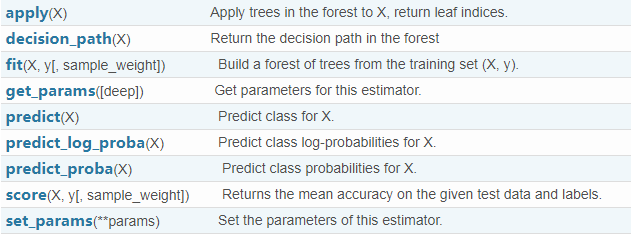五，进行预测的几种常用的方法

1 ） predict_proba(x) : 给出带有概率值的结果。每个点在所有label（类别）的概率和为1.

2） predict(x)： 直接给出预测结果，内部还是调用的predict_proba()。根据概率的结果看哪个类型的预测值最高就是那个类型。

3）predict_log_proba(x)： 和predict_proba基本上一样，只是把结果做了log()处理。

>>> from sklearn.cross_validation import cross_val_score
>>> from sklearn.datasets import make_blobs
>>> from sklearn.ensemble import RandomForestClassifier
>>> from sklearn.ensemble import ExtraTreesClassifier
>>> from sklearn.tree import DecisionTreeClassifier

>>> X, y = make_blobs(n_samples=10000, n_features=10, centers=100,
...     random_state=0)

>>> clf = DecisionTreeClassifier(max_depth=None, min_samples_split=1,
...     random_state=0)
>>> scores = cross_val_score(clf, X, y)
>>> scores.mean()
0.97...

>>> clf = RandomForestClassifier(n_estimators=10, max_depth=None,
...     min_samples_split=1, random_state=0)
>>> scores = cross_val_score(clf, X, y)
>>> scores.mean()
0.999...

>>> clf = ExtraTreesClassifier(n_estimators=10, max_depth=None,
...     min_samples_split=1, random_state=0)
>>> scores = cross_val_score(clf, X, y)
>>> scores.mean() > 0.999
True

六，随机森林分类算法的实现

代码：

#_*_coding:UTF_8_*_

# 导入需要导入的库
import pandas as pd
import  numpy as np
import math
from sklearn.ensemble import RandomForestClassifier
from sklearn.ensemble import ExtraTreesClassifier
from sklearn.tree import DecisionTreeClassifier
from sklearn import model_selection ,metrics
from sklearn.model_selection import cross_val_score
from sklearn.model_selection import train_test_split
import matplotlib.pyplot as plt
import matplotlib as mpl
from sklearn.datasets import make_blobs
import warnings

# 忽略一些版本不兼容等警告
warnings.filterwarnings("ignore")

# 每个样本有几个属性或者特征
n_features = 2
x,y = make_blobs(n_samples=300,n_features=n_features,centers=6)
x_train,x_test,y_train,y_test = train_test_split(x,y,random_state=1,train_size=0.7)

# 绘制样本显示
# plt.scatter(x[:,0],x[:,1],c=y)
# plt.show()

# 传统决策树，随机森林算法 极端随机数的区别
DT = DecisionTreeClassifier(max_depth=None,min_samples_split=2,random_state=0)

RF = RandomForestClassifier(n_estimators=10,max_features=math.sqrt(n_features),
max_depth=None,min_samples_split=2,bootstrap=True)

EC = ExtraTreesClassifier(n_estimators=10,max_features=math.sqrt(n_features),
max_depth=None,min_samples_split=2,bootstrap=False)

# 训练
DT.fit(x_train,y_train)
RF.fit(x_train,y_train)
EC.fit(x_train,y_train)

#区域预测
# 第0列的范围
x1_min,x1_max = x[:,0].min(),x[:,0].max()
# 第1列的范围
x2_min,x2_max = x[:,1].min(),x[:,1].max()
# 生成网格采样点行列均为200点
x1,x2 = np.mgrid[x1_min:x1_max:200j,x2_min:x2_max:200j]
# 将区域划分为一系列测试点用去学习的模型预测，进而根据预测结果画区域
area_sample_point = np.stack((x1.flat,x2.flat),axis=1)

# 所有区域点进行预测
area1_predict = DT.predict(area_sample_point)
area1_predict = area1_predict.reshape(x1.shape)

area2_predict = RF.predict(area_sample_point)
area2_predict = area2_predict.reshape(x1.shape)

area3_predict = EC.predict(area_sample_point)
area3_predict = area3_predict.reshape(x1.shape)

# 用来正常显示中文标签
mpl.rcParams['font.sans-serif'] = [u'SimHei']
# 用来正常显示负号
mpl.rcParams['axes.unicode_minus'] = False

# 区域颜色
classifier_area_color = mpl.colors.ListedColormap(['#A0FFA0','#FFA0A0','#A0A0FF'])
# 样本所属类别颜色
cm_dark = mpl.colors.ListedColormap(['r','g','b'])

# 绘图
# 第一个子图
plt.subplot(2,2,1)

plt.pcolormesh(x1,x2,area1_predict,cmap = classifier_area_color)
plt.scatter(x_train[:,0],x_train[:,1],c =y_train,marker='o',s=50,cmap=cm_dark)
plt.scatter(x_test[:,0],x_test[:,1],c =y_test,marker='x',s=50,cmap=cm_dark)

plt.xlabel('data_x',fontsize=8)
plt.ylabel('data_y',fontsize=8)
plt.xlim(x1_min,x1_max)
plt.ylim(x2_min,x2_max)
plt.title(u'DecisionTreeClassifier: 传统决策树',fontsize=8)
plt.text(x1_max-9,x2_max-2,u'o-------train ; x--------test$') # 第二个子图 plt.subplot(2,2,2) plt.pcolormesh(x1,x2,area2_predict,cmap = classifier_area_color) plt.scatter(x_train[:,0],x_train[:,1],c =y_train,marker='o',s=50,cmap=cm_dark) plt.scatter(x_test[:,0],x_test[:,1],c =y_test,marker='x',s=50,cmap=cm_dark) plt.xlabel('data_x',fontsize=8) plt.ylabel('data_y',fontsize=8) plt.xlim(x1_min,x1_max) plt.ylim(x2_min,x2_max) plt.title(u'RandomForestClassifier: 随机森林算法',fontsize=8) plt.text(x1_max-9,x2_max-2,u'o-------train ; x--------test$')

# 第三个子图
plt.subplot(2,2,3)

plt.pcolormesh(x1,x2,area3_predict,cmap = classifier_area_color)
plt.scatter(x_train[:,0],x_train[:,1],c =y_train,marker='o',s=50,cmap=cm_dark)
plt.scatter(x_test[:,0],x_test[:,1],c =y_test,marker='x',s=50,cmap=cm_dark)

plt.xlabel('data_x',fontsize=8)
plt.ylabel('data_y',fontsize=8)
plt.xlim(x1_min,x1_max)
plt.ylim(x2_min,x2_max)
plt.title(u'ExtraTreesClassifier: 极端随机树',fontsize=8)
plt.text(x1_max-9,x2_max-2,u'o-------train ; x--------test$') # 第四个子图 plt.subplot(2,2,4) y = [] # 交叉验证 score_DT = cross_val_score(DT,x_train,y_train) y.append(score_DT.mean()) score_RF = cross_val_score(RF,x_train,y_train) y.append(score_RF.mean()) score_EC = cross_val_score(EC,x_train,y_train) y.append(score_EC.mean()) print('DecisionTreeClassifier交叉验证准确率为:'+str(score_DT.mean())) print('RandomForestClassifier交叉验证准确率为:'+str(score_RF.mean())) print('ExtraTreesClassifier交叉验证准确率为:'+str(score_EC.mean())) x = [0,1,2] plt.bar(x,y,0.4,color='green') plt.xlabel("0--DecisionTreeClassifier;1--RandomForestClassifier;2--ExtraTreesClassifie", fontsize=8) plt.ylabel("平均准确率", fontsize=8) plt.ylim(0.9, 0.99) plt.title("交叉验证", fontsize=8) for a, b in zip(x, y): plt.text(a, b, b, ha='center', va='bottom', fontsize=10) plt.show() 结果：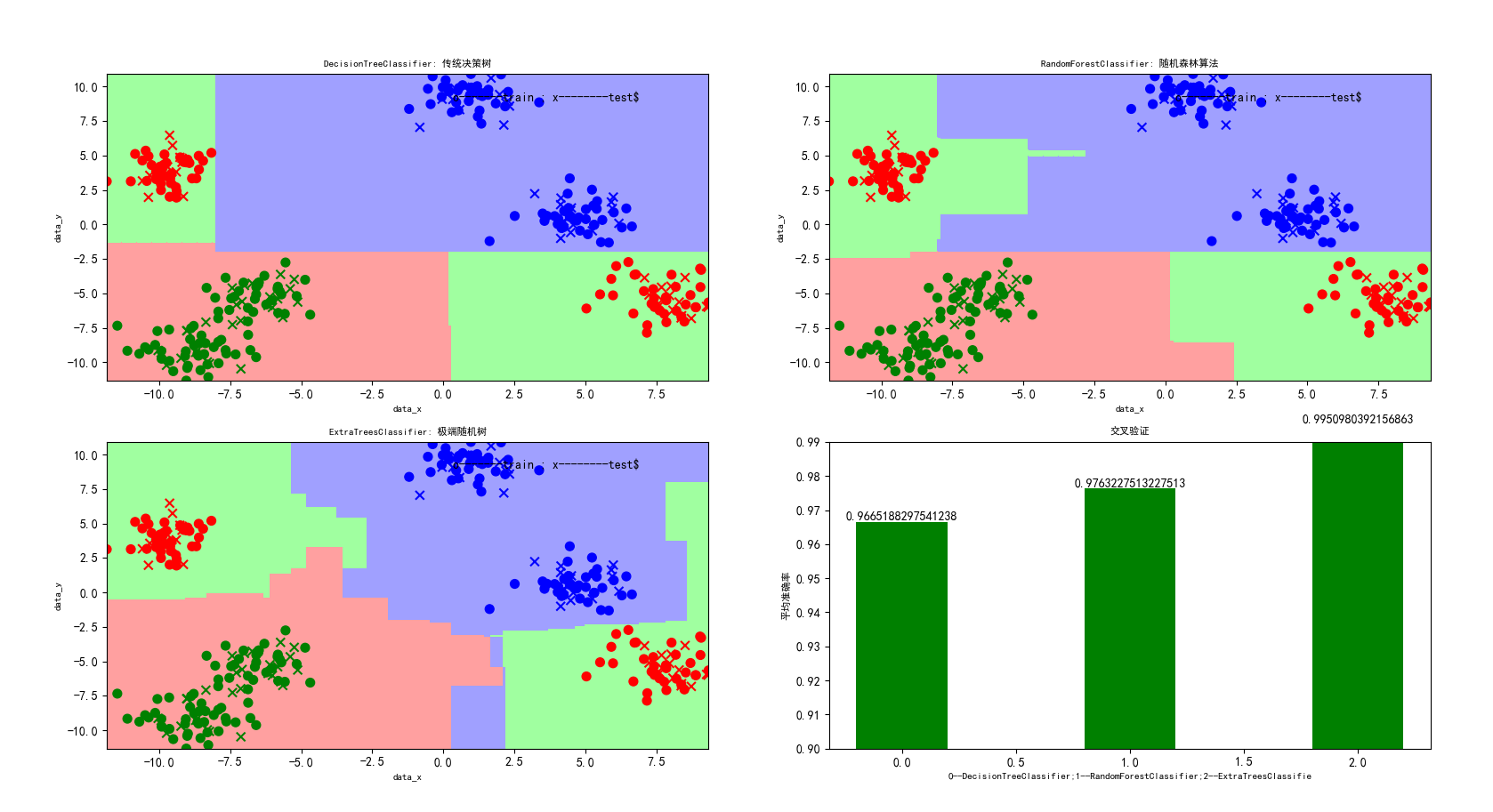七，随机森林回归算法的实现 代码： #随机森林回归 import matplotlib as mpl import numpy as np import warnings import matplotlib.pyplot as plt from sklearn.tree import DecisionTreeRegressor from sklearn.ensemble import RandomForestRegressor from sklearn.ensemble import ExtraTreesRegressor #忽略一些版本不兼容等警告 warnings.filterwarnings("ignore") #产生心状坐标 t = np.arange(0,2*np.pi,0.1) x = 16*np.sin(t)**3 x=x[:, np.newaxis] y = 13*np.cos(t)-5*np.cos(2*t)-2*np.cos(3*t)-np.cos(4*t) y[::7]+= 3* (1 - np.random.rand(9)) #增加噪声，在每数2个数的时候增加一点噪声 #传统决策树线性回归,随机森林回归，极端森林回归 rf1=DecisionTreeRegressor() rf2=RandomForestRegressor(n_estimators=1000) #一般来说n_estimators越大越好，运行结果呈现出的两种结果该值分别是10和1000 rf3=ExtraTreesRegressor() #三种算法的预测 y_rf1 =rf1.fit(x,y).predict(x) y_rf2 =rf2.fit(x,y).predict(x) y_rf3 =rf3.fit(x,y).predict(x) #为了后面plt.text定位 x1_min, x1_max = x[:].min(), x[:].max() x2_min, x2_max = y[:].min(), y[:].max() mpl.rcParams['font.sans-serif'] = [u'SimHei'] #用来正常显示中文标签 mpl.rcParams['axes.unicode_minus'] = False plt.scatter(x, y, color='darkorange', label='data') plt.hold('on') plt.plot(x, y_rf1, color='b', label='DecisionTreeRegressor') plt.plot(x, y_rf2, color='g', label='RandomForestRegressor') plt.plot(x, y_rf3, color='r', label='ExtraTreesRegressor') plt.xlabel('data_x') plt.ylabel('data_y') plt.title('python_machine-learning_RandomForest(n_estimators=1000)-----心状学习') plt.legend() plt.text(x1_max-4, x2_max-1, u'$o---Sample-Point\$')
plt.show()

结果：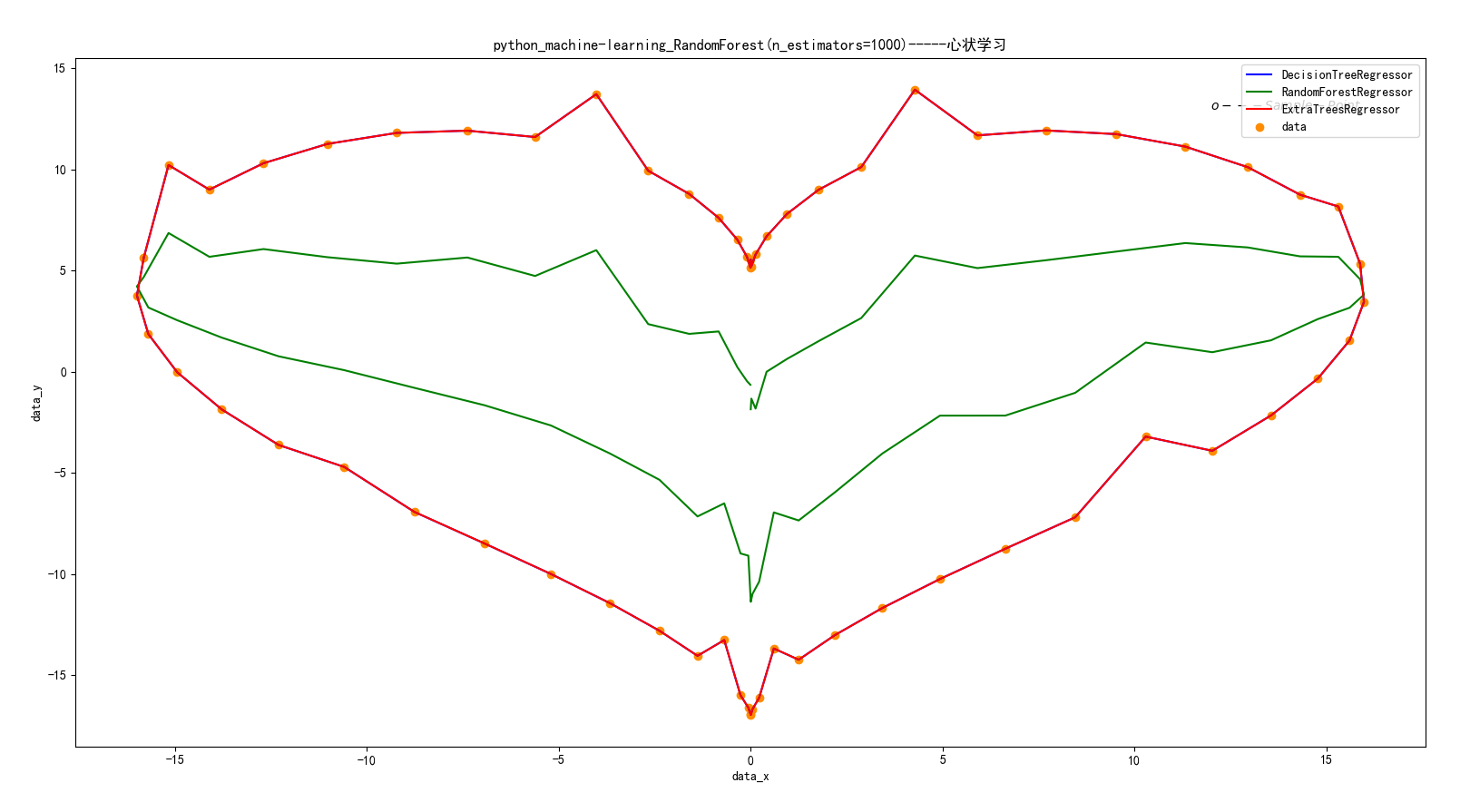八，随机森林分类算法其他机器学习分类算法进行对比

代码：

import numpy as np
import matplotlib.pyplot as plt
from matplotlib.colors import ListedColormap
from sklearn.cross_validation import train_test_split
from sklearn.preprocessing import StandardScaler
from sklearn.datasets import make_moons, make_circles, make_classification
from sklearn.neighbors import KNeighborsClassifier
from sklearn.svm import SVC
from sklearn.tree import DecisionTreeClassifier
from sklearn.naive_bayes import GaussianNB
from sklearn.lda import LDA
from sklearn.qda import QDA

h = .02  # step size in the mesh

names = ["Nearest Neighbors", "Linear SVM", "RBF SVM", "Decision Tree",
"Random Forest", "AdaBoost", "Naive Bayes", "LDA", "QDA"]
classifiers = [
KNeighborsClassifier(3),
SVC(kernel="linear", C=0.025),
SVC(gamma=2, C=1),
DecisionTreeClassifier(max_depth=5),
RandomForestClassifier(max_depth=5, n_estimators=10, max_features=1),
GaussianNB(),
LDA(),
QDA()]

X, y = make_classification(n_features=2, n_redundant=0, n_informative=2,
random_state=1, n_clusters_per_class=1)
rng = np.random.RandomState(2)
X += 2 * rng.uniform(size=X.shape)
linearly_separable = (X, y)

datasets = [make_moons(noise=0.3, random_state=0),
make_circles(noise=0.2, factor=0.5, random_state=1),
linearly_separable
]

figure = plt.figure(figsize=(27, 9))
i = 1
# iterate over datasets
for ds in datasets:
# preprocess dataset, split into training and test part
X, y = ds
X = StandardScaler().fit_transform(X)
X_train, X_test, y_train, y_test = train_test_split(X, y, test_size=.4)

x_min, x_max = X[:, 0].min() - .5, X[:, 0].max() + .5
y_min, y_max = X[:, 1].min() - .5, X[:, 1].max() + .5
xx, yy = np.meshgrid(np.arange(x_min, x_max, h),
np.arange(y_min, y_max, h))

# just plot the dataset first
cm = plt.cm.RdBu
cm_bright = ListedColormap(['#FF0000', '#0000FF'])
ax = plt.subplot(len(datasets), len(classifiers) + 1, i)
# Plot the training points
ax.scatter(X_train[:, 0], X_train[:, 1], c=y_train, cmap=cm_bright)
# and testing points
ax.scatter(X_test[:, 0], X_test[:, 1], c=y_test, cmap=cm_bright, alpha=0.6)
ax.set_xlim(xx.min(), xx.max())
ax.set_ylim(yy.min(), yy.max())
ax.set_xticks(())
ax.set_yticks(())
i += 1

# iterate over classifiers
for name, clf in zip(names, classifiers):
ax = plt.subplot(len(datasets), len(classifiers) + 1, i)
clf.fit(X_train, y_train)
score = clf.score(X_test, y_test)

# Plot the decision boundary. For that, we will assign a color to each
# point in the mesh [x_min, m_max]x[y_min, y_max].
if hasattr(clf, "decision_function"):
Z = clf.decision_function(np.c_[xx.ravel(), yy.ravel()])
else:
Z = clf.predict_proba(np.c_[xx.ravel(), yy.ravel()])[:, 1]

# Put the result into a color plot
Z = Z.reshape(xx.shape)
ax.contourf(xx, yy, Z, cmap=cm, alpha=.8)

# Plot also the training points
ax.scatter(X_train[:, 0], X_train[:, 1], c=y_train, cmap=cm_bright)
# and testing points
ax.scatter(X_test[:, 0], X_test[:, 1], c=y_test, cmap=cm_bright,
alpha=0.6)

ax.set_xlim(xx.min(), xx.max())
ax.set_ylim(yy.min(), yy.max())
ax.set_xticks(())
ax.set_yticks(())
ax.set_title(name)
ax.text(xx.max() - .3, yy.min() + .3, ('%.2f' % score).lstrip('0'),
size=15, horizontalalignment='right')
i += 1

plt.show()

结果：这里随机生成了三个样本集，分割面近似为月形、圆形和线形的。我们可以重点对比一下决策树和随机森林对样本空间的分割：

1）从准确率上可以看出，随机森林在这三个测试集上都要优于单棵决策树，90%>85%，82%>80%，95%=95%；

2）从特征空间上直观地可以看出，随机森林比决策树拥有更强的分割能力（非线性拟合能力）。

九，解决问题：在调用sklearn时出现 Unknown label type: 'unknown' 或者'continuous'

在sklearn模型训练出现如下错误：

‘ValueError: Unknown label type: ‘unknown’

解决方法：以GBDT为例：train_y后加上astype(‘int’)即可

gbdt.fit(train_x,train_y.astype(‘int’))

https://www.jianshu.com/p/a779f0686acc

https://blog.csdn.net/qq547276542/article/details/78304454

https://blog.csdn.net/sun_shengyun/article/details/54618121

https://blog.csdn.net/zjuPeco/article/details/77371645?locationNum=7&fps=1

https://www.cnblogs.com/simpleDi/p/9979818.html

posted @ 2019-02-25 09:04  战争热诚  阅读(30682)  评论(28编辑  收藏  举报